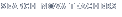Back to Teachers HomeEinstein's Big IdeaClassroom ActivitiesSquaring Off With Velocity

Activity Summary
Students investigate the meaning of c2 in E = mc2 by measuring the energy delivered by an object falling at different velocities. Graphing data leads students to understand that E is proportional to velocity squared, not simply velocity.

Learning Objectives
Students will be able to:

• explain what the c2 in E = mc2 represents.

• state that kinetic energy is the energy of an object in motion.

• illustrate how kinetic energy can be transferred to other objects.

• understand that the energy delivered by an object in motion is proportional to v2, not v.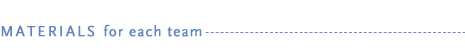• copy of the "Squaring Off With Velocity" student handout (PDF or HTML)
• copy of the "Data Sheet" student handout (PDF or HTML)
• 1 lb flour in a plastic bag
• plastic pan (about shoebox size)
• two 8-oz plastic cups
• four standard-sized (about 1 cm) glass marbles
• plastic ruler
• meter stick
• plastic spoon
• wood dowel or wood barbecue skewer
• felt-tip pen
• graph paper
• newspaper

Background
Light has fascinated scientists for centuries. Galileo Galilei was the first to consider measuring its speed. In 1676, astronomer Ole Roemer made observations of the eclipses of Jupiter's moons to demonstrate that light moved at a very fast—but not infinite—speed. James Clerk Maxwell provided the mathematical backbone for electromagnetism and demonstrated that light was an electromagnetic wave. The squared part of Albert Einstein's equation heralds back to natural philosopher Gottfried Leibniz, who proposed that an object's energy is the product of its mass times its velocity squared, not just its velocity. Emilie du Châtelet further championed his ideas. While many of these scientists were innovative thinkers, determination also played a large part in their achievements. They were willing to challenge widely held beliefs of their day. Their courage and perseverance helped lay the groundwork for Einstein's eventual connection of mass, energy, and the speed of light squared.

In this activity, students use a simple model to investigate the relationship between velocity and energy. Their investigation leads them to conclude that the energy delivered to a system depends on the velocity squared of the impacting body, not simply on the velocity. Students then relate this fact to E = mc2.

The model uses a glass marble as a falling object that impacts a cup of flour. The impact velocity of the marble is a function of the height from which the marble is dropped. The energy released by the falling marble (its potential energy now turned into kinetic energy) is equal to the work done on the flour. The work done on the flour, in turn, is equal to the force (mass x deceleration) it takes to slow the marble down to zero velocity over the distance it penetrates the flour.

Four heights are used-10, 25, 50, and 100 centimeters. Students graph the velocity the marble attains when dropped from these heights against the depth to which the marble penetrates the flour. The depth, in turn, is a measure of the energy that the marble delivers. Students can calculate the impact velocity at these four heights by using the equation: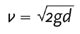where g = the acceleration due to gravity (9.8 m/sec2), and d = the distance the marble falls (in meters)

Key Terms

kinetic energy: Energy of a moving object.

speed: The rate at which an object moves.

velocity: The speed and direction of a moving object.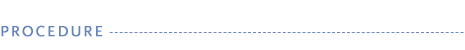1. Assemble all the materials needed for the activity.

2. Write E = mc2 on the board and ask students what the three letters in the equation represent. Emphasize that c stands for a particular constant speed or velocity, that of light in a vacuum.

3. Demonstrate the parts of the apparatus students will use to find the relationship between E and v (a replacement in the model for c). Marbles must be dropped from rest, and the depth to which the marbles penetrate must be measured with a dowel or skewer marked off in centimeters. The depth should be measured from the top of the marble to the top of the flour. The cup must be picked up, and the skewer must be viewed from the side to measure depth accurately. Students should add a half centimeter to their measurements in order to measure to the marble's center of mass.

4. Organize students into teams. Distribute the student handouts and materials. Assign teams to one of the four heights from which to drop marbles. To ensure data reliability, have several teams perform the same measurements multiple times and average the results. As a class data table is going to be made, the more data points the better.

5. Have students place newspaper on the floor, then place their cups filled with flour in the center of a plastic pan. If students run out of clear area in which to drop the marble, have them scoop out the marble(s) with the plastic spoon, use the spoon to refill the cup, tap the base of the cup three times to remove air pockets, and then use the dowel to level the flour to the cup's rim. If students' fingers plunge in to retrieve marbles, they will pack down the flour and their next set of data will be skewed with lower penetrating distances. Results will also be skewed if they leave air pockets in the flour.

6. Ask students how they might find the velocity of the marble as it hits the flour. When they arrive at the correct mathematical strategyhave students calculate the velocity values for the four given heights. (Students will need to convert centimeter drop heights to meters.) Students may notice that doubling the height from which they drop the marble does not double the velocity. If the calculations are too rigorous for students, provide them with the values for velocity (see Activity Answer) and tell students to square them for the v2 column of their table.

7. When student teams are finished, create a class data chart on the board and have students fill it in or have them enter their data into a computer spreadsheet.

8. This is an ideal time to do some data analysis and statistics. Student answers may vary quite a bit. For a given distance, ask students which of the data points are "wrong" and which ones are "right." Discuss the best way to average the numbers so students can graph just one depth for each distance dropped. Cross out the two highest and lowest points (outliers) and average the rest. Have students average all teams' depth results to determine final class averages for each depth. Have students enter the results in the depth column in the "Velocity vs. Energy Data Table" on their "Data Sheet" handout.

9. Discuss factors that may cause data to vary, i.e., non-uniform density of the flour, problems with measuring depth, variations in tick marks, etc. Emphasize that while accuracy is important, measurements may include a degree of error. The goal is to see the pattern the data set (that energy is proportional to velocity squared, not velocity).

10. Tell students they will make two graphs, the first with the y-axis labeled Velocity (meters/second) and the x-axis labeled Energy (depth in centimeters). The second graph will have the y-axis labeled Velocity2 (meters2/second2) and the x-axis labeled Energy (depth in centimeters). Meters are used on the velocity axes to simplify graphing. Note that 0,0 must be used as a data point when drawing the curve.

11. Have students plot the first graph. When students have finished plotting points, review how to interpolate and draw a curve through a set of points instead of drawing "dot to dot," as students will often do. Discuss the fact that a curve shows that the two variables are not directly proportional.

12. Now have students plot their second graph in which the velocity is squared. After students finish their second graph, help them draw a straight line through as many points as possible. They should try to have roughly the same number of points on either side of their lines. Then have student teams answer the questions on their "Data Sheet" handout. Review answers as a class. What is the most noticeable difference between the two graphs?

13. As an extension, have students useto calculate from what heights marbles would need to be dropped to double the velocity for each height (beginning with the 10-centimeter height). Have the students repeat the experiment at this new set of heights (you may need to use very wide-mouth cups in order for students to hit the target flour). Extend the heights above the flour to 2 meters. Stop taking data when the marble hits the bottom of the cup. Direct students to plot this new set of data, compare it to their previous graphs, and find the slopes for each line. Are they the same? Have students explain their results.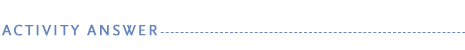Marbles dropped from different heights accelerate toward the surface of the flour, increasing their velocity and kinetic energy as they fall. The kinetic energy that the marble has gained is then transferred to the flour as it plunges in. The depth that the marble reaches in the flour is a measure of the kinetic energy that is transferred to the flour (the energy deforms the flour and makes the marble crater).

Student results may vary due to differences in flour density and errors in dropping the marble from prescribed heights. When reviewing the table with students, it would be best to eliminate the two lowest and highest values for each height and average the rest. The summary data plotted should reveal that energy is proportional to velocity squared.

Sample Results

Velocity vs. Energy Data Table

 Distance (cm) v (m/sec) v2 (m2/sec2) Depth (cm) 0 0 0 0 10 1.4 2.0 0.5 25 2.2 4.9 1.3 50 3.1 9.8 2.4 100 4.4 19.6 4.1

The average error of the depth data was about +/- .5 cm.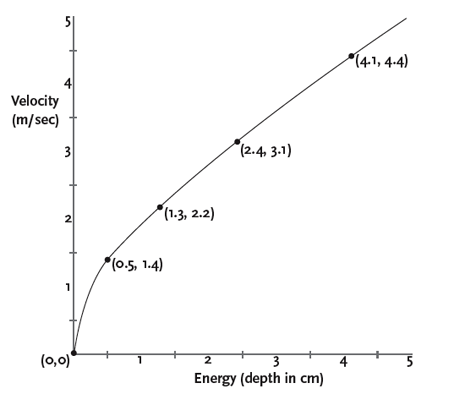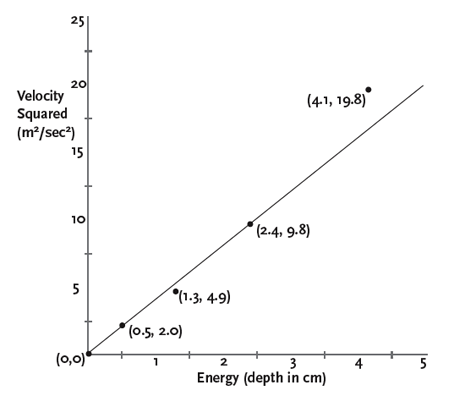Student Handout Questions

1. What is the shape of your Velocity vs. Energy graph? The shape of the graph is a curve.

2. What is the shape of your Velocity2 vs. Energy graph? The shape of the graph should be close to a straight line.

3. If a straight line on a graph indicates a direct relationship, is energy (measured by depth) directly proportional to velocity or velocity squared? The Velocity2 graph appears to show that energy is proportional to velocity squared, not velocity.

4. Explain why Albert Einstein wrote E = mc2 instead of E = mc. Students may forget that the c is simply the velocity of light. Einstein wrote c2 because E is directly proportional to velocity squared, not velocity.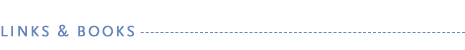Web Sites

NOVA—Einstein's Big Idea
www.pbs.org/nova/einstein
Hear top physicists explain E = mc2, discover the legacy of the equation, see how much energy matter contains, learn how today's physicists are working with the equation, read quotes from Einstein, and more on this companion Web site.

Answers several questions related to light and E = mc2.

The Electromagnetic Spectrum
imagers.gsfc.nasa.gov/ems/waves3.html
Describes the electromagnetic spectrum and includes information on visible light.

The Ultimate Physics Resource Site
serendip.brynmawr.edu/local/IIT/projects/Glasser.html

Books

40 Low-Waste, Low-Risk Chemistry Experiments
by David Dougan. Walch Publishing, 1997.
Includes introductory labs on measurement, density, temperature, relative mass, and more.

Energy by Jack Challoner. Dorling Kindersley, 1993.
Surveys various sources of energy and the ways in which they have been harnessed.

Light
by David Burnie. Dorling Kindersley, 1999.
Explains many aspects of visible light and other forms of electromagnetic energy.

Stop Faking It!: Light
by William C. Robertson. NSTA Press, 2003.
Provides information and activities to help teachers and students understand light.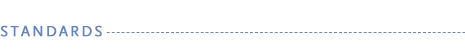The "Messing With Mass" activity aligns with the following National Science Education Standards (see books.nap.edu/html/nses) and Principles and Standards for School Mathematics (see standards.nctm.org/document/index.htm).

Science Standard

Physical Science

• Transfer of energy

Mathematics Standard
Measurement

Science Standard

Physical Science

• Conservation of energy and the increase in disorder

Mathematics Standard
Measurement

Classroom Activity Author

Jeff Lockwood taught high school astronomy, physics, and Earth science for 28 years. He has authored numerous curriculum projects and has provided instruction on curriculum development and science teaching methods for more than a decade.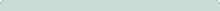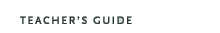Einstein's Big IdeaOriginal broadcast:October 11, 2005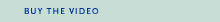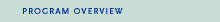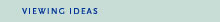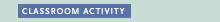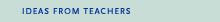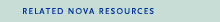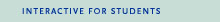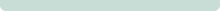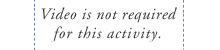The need for c c is necessary in Einstein's equation because whenever part of any piece of matter is converted to pure energy, the resulting energy is by definition moving at the speed of light. Pure energy is electromagnetic radiation—whether light or X-rays or whatever—and electromagnetic radiation travels at a constant speed of about 300,000 kilometers per second. The speed of light must be squared because of the nature of energy. When something is moving four times as fast as something else, it doesn't have four times the energy but rather 16 times the energy—in other words, that figure is squared. So the speed of light squared is the conversion factor that determines just how much energy lies within any type of matter.
 Major funding for NOVA is provided by Google. Additional funding is provided by the Corporation for Public Broadcasting, and public television viewers.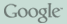Major funding for Einstein's Big Idea is provided by the National Science Foundation. Additional funding is provided by the Alfred P. Sloan Foundation, and the U.S. Department of Energy. This material is based upon work supported by the National Science Foundation under Grant No. 0407104. Any opinions, findings, and conclusions or recommendations expressed in this material are those of the author(s) and do not necessarily reflect the views of the National Science Foundation.